# RBSE Maths Class 11 Chapter 8: Important Questions and Solutions

RBSE Solutions for Class 11 Maths Chapter 8 – Binomial theorem are available here. The important questions and solutions of Chapter 8, available at BYJU’S, contain detailed explanations to every problem, making a student’s life easy. All these important questions are based on the new pattern prescribed by the RBSE. Students can also get the syllabus and textbooks on RBSE Class 11 solutions.

Chapter 8 of the RBSE Class 11 Maths will help the students to solve problems related to the binomial expression, binomial theorem for a positive index, important terms of the binomial theorem, general term in binomial expression, properties of binomial coefficients, binomial theorem for a rational index, important expansions, applications of the binomial theorem, the sum of series by binomial theorem. After referring to these important questions and solutions, you can refer to 2020-2021 solutions for the chapter here.

### RBSE Maths Chapter 8: Miscellaneous Questions and Solutions

Question 1: The number of terms in the expansion of ((a / x) + bx)12 are

(A) 11

(B) 13

(C) 10

(D) 14

Solution:

The terms of the right-hand side of the expansion of (x + a)n are finite for the positive values of x and the number of terms is (n + 1).

So, the value of n is 12 in the given expression.

Hence, number of total terms = 12 + 1 = 13.

Hence, option (B) is correct.

Question 2: The 7th term in the expansion of ((1 / 2) + a)8 is

(A) 8C7 (1 / 2) a7

(B) 8C7 (1 / 2) a

(C) 8C6 (1 / 2)2 (a)6

(D) 8C6 (1 / 2)6 a2

Solution:

The 7th term in the expansion of ((1 / 2) + a)8 is

T7 = T6+1

= 8C6 (1 / 2)8-6 a6

= 8C6 (1 / 2)2 (a)6

Hence, option (C) is correct.

Question 3: If in the expansion of (1 + x)18, coefficients of (2r + 4)th and (r – 2)th terms are equal then value of r is:

(A) 5

(B) 6

(C) 7

(D) 8

Solution:

In the expansion of (1 + x)18 coefficients of (2r + 4)th and (r – 2)th terms are 18C2r+3 and 18Cr–3 respectively.

These are equal, then, 18C2r+3 = 18Cr–3

⇒ 2r + 3 = r – 3

2r + 3 = 18 – (r – 3)

For using the statement if

nCr = nCp or nCn-p

⇒ 2r – r = -3 – 3

2r + 3 = 18 – r + 3

⇒ r = -6 or r = 6 r is a natural number, so r = – 6 is not possible, then x = 6.

Hence, option (B) is correct.

Question 4: If in the expansion of (a + b)n and (a + b)n+3 ratio of 2nd and 3rd, 3rd and 4th terms are equal, then value of n is :

(A) 5

(B) 6

(C) 3

(D) 4

Solution:

In the expansion of (a + b)n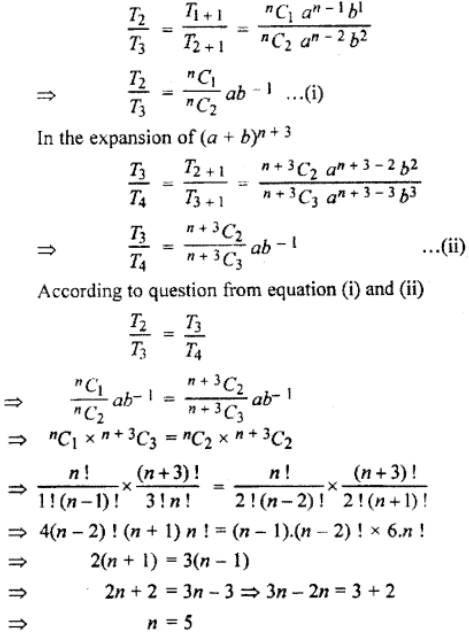Hence, option (A) is correct.

Question 5: If in the expansion of (1 + x)2n, coefficient of 3rd and (r + 2)th term are equal, then :

(A) n = 2r

(B) n = 2r – 1

(C) n = 2r + 1

(D) n = r + 1

Solution:

In the expansion of (1 + x)2n, 3rd term = 2 nC3 –1 and (r + 2)th term = 2nCr+1

According to the question, 2nC3r–1 = 2nCr+1

Now, 3r – 1 = r + 1

3r – 1 = 2n – r – 1

⇒ 3r – r – I + 1

3r + r = 2n – 1 + 1

⇒ 2r = 2 or 4r = 2n

⇒ r =1

2r = n

Hence, option (A) is correct.

Question 6: Find the value of 30C1 + 30C2 + 30C3 +… + 30C30.

Solution:

(1 + x)n = nC0 + nC1x + nC2x2 + nC3x3 + ….

Putting x = 1

(1 + 1)n = nC0 + nC1x + nC2x2 + nC3x3 + …….. nCn or

2n = nC0 + nC1x + nC2x2 + nC3x3 + …….. nCn

Here, putting n = 30.

230 = 1 + 30C1 + 30C2 + 30C3 +… + 30C30

⇒ 1+ 30C1 + 30C2 + 30C3 +… + 30C30 = 230

30C1 + 30C2 + 30C3 +… + 30C30 = 230 – 1

Question 7: Find the middle term in the expansion of [(a / x) + (x / a)]10.

Solution:

Here, the middle term is [(10 / 2) + 1]th term = 6th term.

T6 = T5+1

= 10C5 (a / x)10-5 (x / a)5

= 10C5 (a / x)5 (x / a)5

= 252 * (1)

= 252

### RBSE Maths Chapter 8: Exercise 8.1 Textbook Important Questions and Solutions

Question 1: Expand each expression in the following questions.

[i] (2 – x)3

[ii] (3x + 2y)4

[iii] [√(x / a) – √9 / x]6

Solution:

[i] (2 – x)3

= 3C0 (2)3 + 3C1 (2)2 (-x) + 3C2 (2) (-x)2 + 3C3 (-x)3

= 1 * 8 + 3 * 4 * (-x) + 3 * 2 * x2 + 1 * (-x)3

= 8 – 12x + 6x2 – x3

[ii] (3x + 2y)4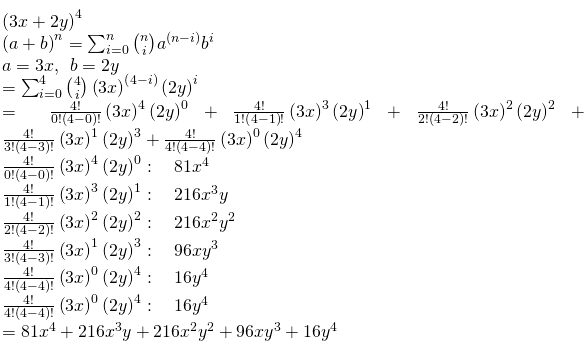[iii] [√(x / a) – √9 / x]6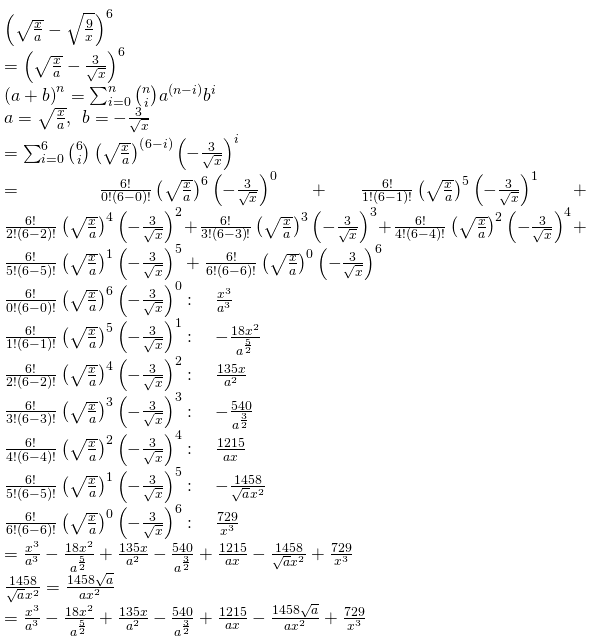Question 2: Using the binomial theorem, find the value of (1.1)6.

Solution:

(1.1)6

= (1 + 0.1)6

= 6C0(1)6 (0.1)0 + 6C1 (1)5 (0.1)1 + 6C2 (1)4 (0.1)2 + 6C3 (1)3 (0.3)3 + 6C4 (1)2 (0.1)4 + 6C5 (1)1 (0.1)5 + 6C6 (1)0 (0.1)6

= (1 * 1 * 1) + (6 * 1 * 0.1) + {15 * 1 * (0.1)2} + {20 * 1 * (0.1)3} + {15 * 1 * (0.1)4} + {6 * 1 * (0.1)5} + {1 * 1 * (0.1)6}

= 1 + 6 * 0.1 + 15 * (0.1)2 + 20 * (0.1)3 + 15 * (0.1)4 + 6 * (0.1)5 + (0.1)6

= 1 + 0.6 + 15 * 0.01 + 20 * 0.001 + 15 * 0.0001 + 6 * 0.00001 + 0.000001

= 1 + 0.6 + 0.15 + 0.020 + 0.0015 + 0.00006 + 0.000001

= 1.771561

### RBSE Maths Chapter 8: Exercise 8.2 Textbook Important Questions and Solutions

Question 1: In the following expansions, find the terms as stated.

[i] 5th term of (a + 2x3)17

[ii] 9th term of ([x / y] – [3y / x2])17

[iii] 6th term of ([2 / √x] – [x2 / 2])9

Solution:

[i] 5th term of (a + 2x3)17

The rth term in an expansion of (a + x)n is given by nCr−1 an+1−r xr−1.

Similarly, the fifth term in the expansion of (a + 2x3)17 is given by

= 17C4 a13 (2x)4

=  * 16 a13 * x12

= 38080 a13 x12

[ii] 9th term of ([x / y] – [3y / x2])17

Tr+1 = nCr an-r br

a = [x / y], b = [3y / x2], n = 12, r = 8

9th term = T9 = T8+1

T9 = 12C8 [x / y]12-8 [3y / x2]8

= 495 * [x / y]4 [3y / x2]8

= 495 * 6561 * x4-16 y-4+8

= [3247695 / x12] y4

[iii] 6th term of ([2 / √x] – [x2 / 2])9

Tr+1 = nCr an-r br

a = [2 / √x], b = [- x2 / 2], n = 9, r = 5

6th term = T6 = T5+1

T6 = 9C5 [2 / √x]9-5 [- x2 / 2]5

= 126 * [2 / √x]4 [- x2 / 2]5

= – [2016 / 32] * x10-[4/2]

= –  x8

Question 2: Find the coefficient of the following.

(i) x-7 in the expansion of [ax – (1 / bx2)]8

(ii) x4 in the expansion of [(x4 + [1 / x3]]15

(iii) x6 in the expansion (a – bx2)10

Solution:

(i) x-7 in the expansion of [ax – (1 / bx2)]8

[ax – (1 / bx2)]

(r + 1)th term of [ax – (1 / bx2)]8

Tr+1 = 8Cr (ax)8-r [-1 / bx2]r

Tr+1 = (-1)r 8Cr a8-r x8-r [1 / br x2r]

Tr+1 = (-1)r 8Cr [a8-r / br] x8-r-2r

Tr+1 = (-1)r 8Cr [a8-r / br] x8-3r

In this term for coefficient of x-7 power of x should be

-7 = 8 – 3r = – 7

Hence,

8 – 3r = – 7

⇒ -3r = – 7 – 8 = – 15

r = 5

Hence, coefficient of x-7 in the expansion is

(-1)5 8C5 [a8-5 / b5] x8-3(5)

= [- 56a3 / b5]

(ii) x4 in the expansion of [(x4 + [1 / x3]]15

[(x4 + [1 / x3]]

(r + 1)th term of [(x4 + [1 / x3]]15

Tr+1 = 15C4 (x4)15-r [1 / x3]r

Tr+1 = 15Cr x60-4r [1 / x3]r

Tr+1 = 15Cr x60-4r-3r

Tr+1 = 15Cr x60-7r

In this term for coefficient of x4 power of x should be

4 = 60 – 7r = -56

Hence,

8 – 3r = – 7

r = 8

Hence, the coefficient of x4 in the expansion is 6435.

(iii) x6 in the expansion (a – bx2)10

(a – bx2)

(r + 1)th term of (a – bx2)10

Tr+1 = 10Cr (a)10-r [– bx2]r

Tr+1 = 10Cr a10-r [-1]r br x2r

Tr+1 = (-1)r 10Cr a10-r br x2r

In this term for coefficient of x6 power of x should be

2r = 6

r = 3

Hence, coefficient of x6 in the expansion is

(-1)3 10C3 [a10-3 b3]

= [- 120a7 b3]

Question 3: In the following expansions, find the term independent of x.

[i] [x – (1 / x2)]12

[ii] [√x – (3 / x2)]10

[iii] [(√x / 3] + (3 / 2x2)]10

[iv] [x2 – (1 / x2)]10

Solution:

[i] [x – (1 / x2)]12

(r + 1)th term of [x – (1 / x2)]12

Tr+1 = 12Cr (x)12-r [(1 / x2)]r

Tr+1 = (-1)r 12Cr x12-r-2r

Tr+1 = (-1)r 12Cr x12-3r

For the term independent of x,

x12-3r = x0

12 – 3r = 0

12 = 3r

12 / 3 = r

r = 4

Hence, the term independent of x is

(-1)4 12C4

= 495

[ii] [√x – (3 / x2)]10

(r + 1)th term of [√x – (3 / x2)]10

Tr+1 = 10Cr (√x)10-r [- (3 / x2)]r

Tr+1 = 10Cr x[10-r]/2 (-1)r 3r x-2r

Tr+1 = (-1)r 10Cr 3r x[10-r]/2 – [2r]

For the term independent of x,

x[10-r]/2 – [2r] = x0

{[10 – r] /  – [2r]} = 0

10 – r – 4r = 0

10 – 5r = 0

r = 2

Hence, the term independent of x is

(-1)2 10C2 32

= 45 * 9

= 405

[iii] [(√x / 3] + (3 / 2x2)]10

(r + 1)th term of [(√x / 3)] + (3 / 2x2)]10

Tr+1 = 10Cr (√x / 3)10-r (3 / 2x2)r

Tr+1 = 10Cr (x / 3)[5-r/2] (3 / 2)r x-2r

Tr+1 = 10Cr (1 / 3)5-r/2 (3 / 2)r x5-(r/2)-2r

(r + 1)th term is constant, that is the power of x should be 0.

5 – (r / 2) – 2r = 0

5 = 5r / 2

r = 2

The constant term = 10C2 (1 / 3)5-(2/2) (3 / 2)2 x5-1-4

= 10C2 (1 / 3)4 (3 / 2)2

= 5 / 4

[iv] [x2 – (1 / x2)]10

(r + 1)th term of [x2 – (1 / x2)]10

Tr+1 = 10Cr (x2)10-r [- (1 / x2)]r

Tr+1 = (-1)r 10Cr x20-2r-2r

Tr+1 = (-1)r 10Cr x[20-4r]

For the term independent of x,

x[20-4r] = x0

20 – 4r = 0

20 = 4r

20 / 4 = r

5 = r

Hence, the term independent of x is

(-1)5 10C5

= -252

Question 4: Find the middle term in the following expansions.

[i] ([x / 2] + 2y)6

[ii] [3a – (a3 / 6)]9

[iii] (x + (1 / x))2n

[iv] [3x – (2 / x2)]15

Solution:

[i] ([x / 2] + 2y)6

The middle term in the expansion of ([x / 2] + 2y)6. In the expansion of ([x / 2] + 2y)6, the middle term will be T[n/2 + 1]th term where n = 6 which is an even number.

The middle term T[n/2 + 1] = T4

T4 = T3+1

= 6C3 [x / 2]6-3 (2y)3

= 20 * [x3 / 23] * 23 * y3

= 20 x3 y3

[ii] [3a – (a3 / 6)]9

Here n = 9, which is an odd number.

The middle term is {[(n + 1) / 2] + }th term and {(n + 1) / 2}th term.

Here the middle term is 5th and 6th term.

Now, Tr+1 = 9Cr (3a)9-r (-a3 / 6)r

Put r = 4,

T5 = 9C4 (3a)9-4 (-a3 / 6)4

= 126 * 35 * a5 * [a12 / 24 34]

= [189 / 8] a17

Put r = 5,

T6 = 9C5 (3a)9-5 (-a3 / 6)5

= -126 / 96 [a19]

= [-21 / 16] a19

The two middle terms are [189 / 8] a17 and [-21 / 16] a19.

[iii] (x + (1 / x))2n

Here n = 2n, which is an even number.

The middle term is {[(2n) / 2] + }th term and {(n + 1)}th term.

Now, Tr+1 = 2nCr (x)2n-r (1 / x)r

Put r = n,

Tn+1 = 2nCn (x)2n-n (1 / xn)

= {(2n)! / [n! * n!]} * xn * (1 / xn)

= (2n)! / (n!)2

The middle term is (2n)! / (n!)2.

[iv] [3x – (2 / x2)]15

Here n = 15, which is an odd number.

The middle term is {[(n + 1) / 2] + }th term and {(n + 1) / 2}th term.

Here the middle term is 8th and 9th term.

Now, Tr+1 = 15Cr (3x)15-r (-2 / x2)r

Put r = 7,

T8 = 15C7 (3x)15-7 (-2 / x2)7

= [6435 * 38 * (-1)7 * 27 * x8] / x14

= [-6435 * 38 * 27] / x6

Put r = 8,

T9 = 15C8 (3x)15-8 (-2 / x2)8

= [6435 * 38 * 28 * x7] / x16

= [6435 * 37 * 28] / x9

The two middle terms are [-6435 * 38 * 27] / x6 and [6435 * 37 * 28] / x9.

Question 5: Prove that if n is even, then in the expansion of (1 + x)n, coefficient of the middle term will be {[1. 3. 5…… (n – 1)] / [2. 4. 6 ……. n]} * 2n. If n is odd, then the coefficient of both the middle term will be {[1. 3. 5…… (n)] / [2. 4. 6 ……. (n + 1)]} * 2n.

Solution:

If n is even in (1 + x) term we take (1 + x)2n in place of (1 + x)n then ‘n’ = 2n is also even.

Now, the middle term = [(2n / 2) + 1]th term = (n + 1)th term.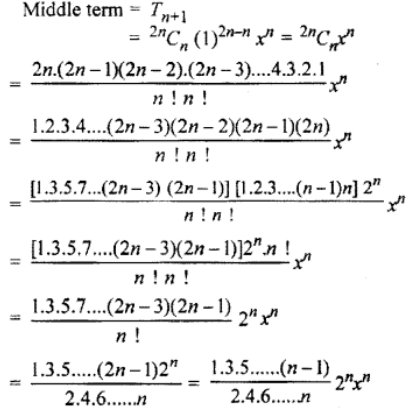Similarly, if n is odd, then we take (1 + x)2n+1 in place of (1 + x)n , then ‘n’ = 2n + 1 is also odd. Now middle term are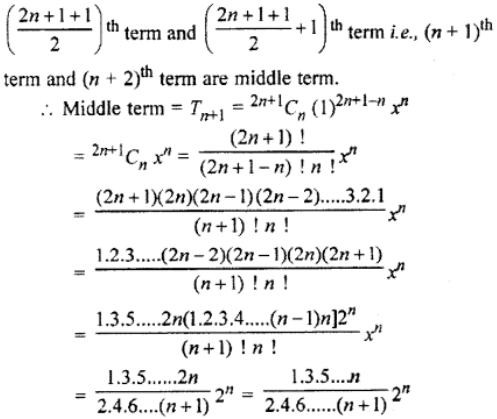Question 6: If in the expansion of (ax + (1 / bx))11, the coefficient of x7 and x-7 are equal, then prove that ab – 1 – 0.

Solution:

(r + 1)th term in the expansion of (ax + (1 / bx))11 is given by

Tr+1 = 11Cr (ax)11-r (1 / bx)r

= 11Cr [(a)11-r / br ] (x)11-r-r

= 11Cr [(a)11-r / br ] (x)11-2r

In this term, the coefficient of x7 in the expansion of

11C2 [(a)11-r / br ]

= 55 * [a9 / b2] —– (1)

Similarly, for the coefficient of x-7,

-2r = -7 – 11

-2r = -18

r = 18 / 2

r = 9

The coefficient of x-7 in the expansion of

55 * [a9 / b2] = 55 * [a2 / b9] [a9 / b9] / [a2 / b2] = 1

(ab)7 = 1

ab = 1

ab – 1 = 0

Question 7: In the expansion of (1 + y)n if coefficients of 5th, 6th and 7th terms are in A.P., then find the value of n.

Solution: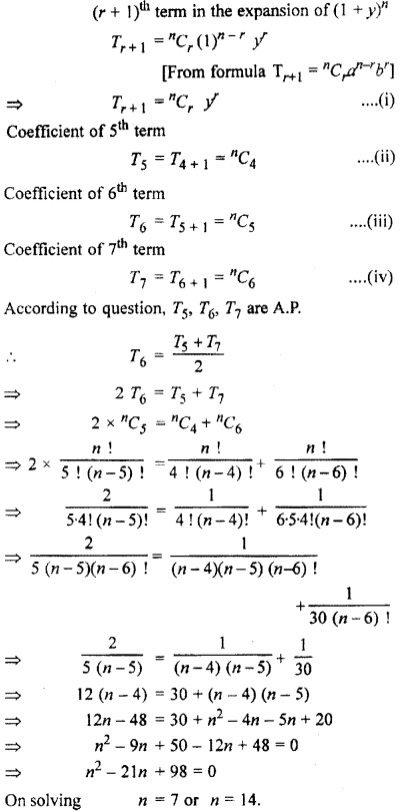Question 8: In the binomial expansion of (x – a)n, the second, third and fourth terms are 250, 720 and 1080, respectively. Find x, a and n.

Solution:

Second term in expansion of (x + a)n

T2 = nC1 xn-1 a

According to the question,

nC1 xn-1 a = 240 —- (1)

Similarly,

T3 = nC2 xn-2 a2 = 720 —- (2)

T4 = nC3 xn-3 a3 = 1080 —- (3)

On dividing (2) from (1), we get

nC2 xn-2 a2 / nC1 xn-1 a = 720 / 240

[(n – 1)! / (n – 2)!] * [a / x] = 6

[a / x] = 6 / n – 1 —- (4)

On dividing (3) by (2), we get

[a / x] = 9 / 2(n – 2) —- (5)

From (4) and (5), we get

6 / n – 1 = 9 / 2(n – 2)

n = 5

From 5x4a = 240 and from (4)

a / x = 3 / 2

On solving both the equations, we get

x = 2, a = 3.

Question 9: If in the expansion of (1 + a)n coefficient of three consecutive terms is in ratio 1: 7: 42, then find the value of n.

Solution:

Let in the expansion of (1 + x)n three consecutive terms are (r – 1 )th term, rth term and (r + 1)th term.

Here, (r – 1)th term = nCr – 2ar-2 and its coefficient, = nCr – 2

Similarly, coefficient of rth term and (r + 1)th term is nCr -1 and nCr respectively.

Now, the given ratio of the coefficients is 1: 7: 42.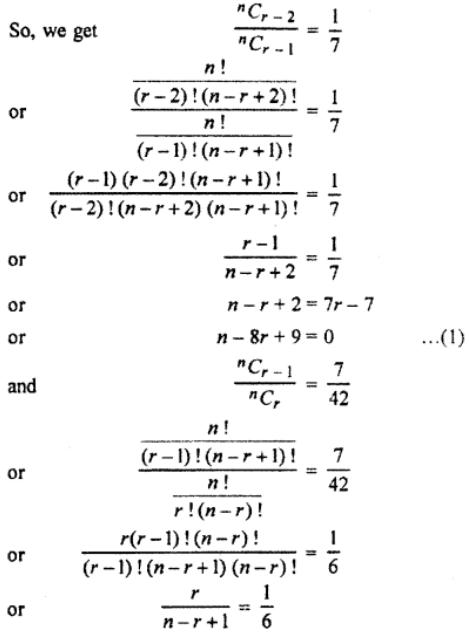6r = n – r + 1

n – 7r + 1 = 0 …….(2)

On solving (1) and (2), we get n = 55.

### RBSE Maths Chapter 8: Exercise 8.3 Textbook Important Questions and Solutions

Question 1: If C0, C1, C2,… Cn are coefficients of expansion (1 + x)n then find the value of :

(i) 8C1 + 8C2 + 8C3 + … + 8C8

(ii) 8C1+ 8C3 + 8C5 + 8C7

Solution:

(i) 8C1 + 8C2 + 8C3 + … + 8C8

We know that

nC0 + nC1+ nC2 + … + nCn = 2n

Putting n = 8

8C0 + 8C1 + 8C2 + 8C3 + … + 8C8 = 28

⇒ 1 + 8C1 + 8C2 + 8C3 + … + 8C8 = 28

8C1 + 8C2 + 8C3 + … + 8C8 = 28 – 1 = 255.

(ii) 8C1+ 8C3 + 8C5 + 8C7

We know that 8C1+ 8C3 + 8C5 + ………. = 2n-1

Putting n = 8

8C1+ 8C3 + 8C5 + 8C7 = 28 -1

= 27

= 128.

Question 2: If C0, C1, C2,… Cn are coefficients of expansion (1 + x)n then prove that:

C0 + 3C1 + 5C2 + … + (2n + 1) Cn = (n + 1)2n.

Solution:

C0 + 3C1 + 5C2 + … + (2n + 1) Cn = (n + 1)2n

= nC0 + (2 + 1) nC1 + (4 + 1) nC2 + … (2n + 1) nCn

= nC0 + (2 * nC1 + nC1 + (4 * nC2 + nC2) + … (2n * nCn + nCn)

= 2 * nC1 + 4 * nC2 + 6 * nC3 + … + 2n * nCn + (nC0 + nC1 + nC2 + nC3 + … nCn)

= 2 * [nC1 + 2 nC2 + 3 * nC3 +… + nCn] + (1 + 1)n

= 2 [n + ((2n(n – 1) / [2!]) + (3 * [(n) (n – 1) (n – 2)] / 3!) + ……. n terms] + 2n

= 2n [1 + (n – 1) + (n(n – 1) / [2!]) + ……. n terms] + 2n

= 2n [1 + 1]n-1 + 2n

= 2n * 2n-1 + 2n

= n * 2n + 2n

= (n + 1) * 2n

Question 3: Prove that C0C2 + C1C3 + C2C4 + … + Cn-2 Cn= [2n!] / [n – 2]! [n + 2]!.

Solution:

From Binomial theorem (1 + x)n = C0 + C1x + C2x2 + … + Crxr + … + Cnxn … (i)

Again (x + 1)n = C0xn + C1xn-1 + C2xn-2 + …+ Crxn – r + …+ Cn-1x + Cn …(ii)

Multiplying equation (i) and (ii) we get

(1 + x)2n = [C0 + C1x + C2x2 + … + Crxr + … + Cnxn ] * [C0xn + C1xn-1 + C2xn-2 + …+ Crxn – r + …+ Cn-1x + Cn …]

Comparing coefficient of xn – r on both sides, we get

C0Cr + C1Cr+1 + C2Cr +2 + … + Cn-rCn

= 2nCn-r = [2n]! / [(n – r)! (2n – n + r)!]

= [2n]! / [(n – r)! (n + r)!] —– (iii)

Putting r = 2 in equation (iii),

C0C2 + C1C3 + C2C4 + … + Cn-2Cn = 2nCn-2 = [2n!] / [n – 2]! [2n – n + 2]!

= [2n!] / [n – 2]! [n + 2]!

Question 4: C0 + 2C1 + 4C2 + 6C3 + … + 2nCn = 1 + n2n.

Solution:

L.H.S.

= C0 + 2C1 + 4C2 + 6C3 + … + 2nCn

= C0 + 2[C1 + 2C2 + 3C3 + … + nCn]

= 1 + 2[n + [2n (n – 1) / 2!] + [3n (n – 1) (n – 2) / 3!] + ……. + n

= 1 + 2n [1 + (n + 1) + (n – 1) (n – 2) / 2! + ….. + 1]

= 1 + 2n (1 + 1)n-1

= 1 + 2n * 2n-1

= 1 + n * 2n-1+1

= 1 + n * 2n

Question 5: [1 + (C1 / C0)] + [1 + C2 / C1] + [1 + C3 / C2] …….. + [1 + Cn / Cn-1] = [n + 1]n / n!.

Solution: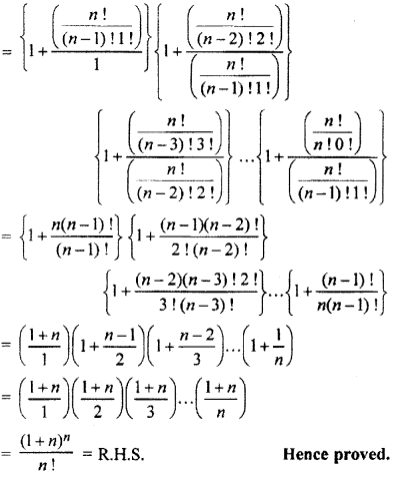Question 6: If expansion of (1 + x – 2x2)6 is denoted by 1 + a1x + a2x2 + a3x3 + … + a12x12 then prove that a2 + a4 + a6 + … + a12 = 31.

Solution:

(1 + x – 2x2)6 = 1 + a1x + a2x2 + a3x3 + … + a12x12 ……… (i)

Putting x = – 1 in equation (i)

{1 + 1 – 2(1)2}6 = 1+ a1 + a2 + a3 + … + a12

⇒ (2 – 2)6 = 1 + a1 + a2 + a3 + … + a12

⇒ 1 + a1 + a2 + a3 + … + a12 = 0 … (ii)

Putting x = – 1 in equation (i)

{(1 – 1 – 2(-1)2}6 = 1 – a1 + a2 + a3 + … + a12

⇒ (-2)6 = 1 – a1 + a2 + a3 + … + a12

⇒ 1 – a1 + a2 + a3 + … + a12 = 64 … (iii)

Adding equation (ii) and (iii) we get

⇒ 2 + 2a2 + 2a4 + … + a12 = 64

⇒ 2(1 + a2 + a4 + … + a12 = 64

⇒ 1 + a2 + a4 + … + a12 = 64 / 2 = 32

⇒ a2 + a4 + … + a12 = 32 – 1 = 31

Hence, a2 + a4 + … + a12 =31.

### RBSE Maths Chapter 8: Exercise 8.4 Textbook Important Questions and Solutions

Question 1: Expand the binomial [1 + x2]-2 upto fourth term.

Solution:

Expansion up to four terms of [1 + x2]-2

[1 + x2]-2 = 1 + (-2) x2 + [(-2) (-2 – 1) (x2)2 / [2!]] + [(-2) (-2 – 1) (-2 – 2) (x2)3 / [3!]]

= 1 – 2x2 + [6x4 / 2!] + [(-2) (-3) (-4) x6] / 3!

= 1 – 2x2 + [6x4 / 2!] – [24x6 / 3!]

= 1 – 2x2 + [6x4 / 2 * 1] – [24x6 / 3 * 2 * 1]

= 1 – 2x2 + 3x4 – 4x6

Question 2: Find the seventh term of the expansion (1 + x)5/2.

Solution: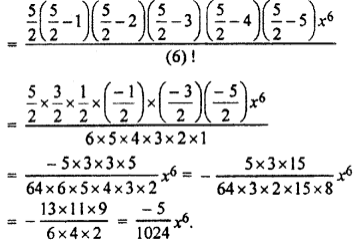Question 3: Find the general term of the expansion of (a3 – x3).

Solution: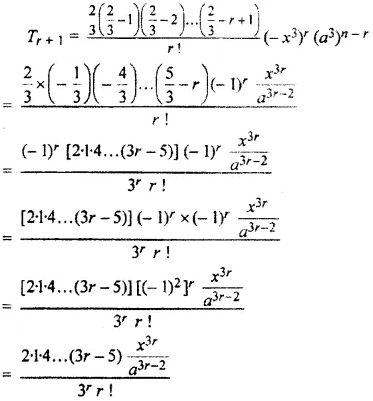Question 4: If x < 3, find the coefficient of (3 – x)-8 in the expansion of (3 – x)-5.

Solution:

(x + 1)th term in the expansion of (3 – x)-8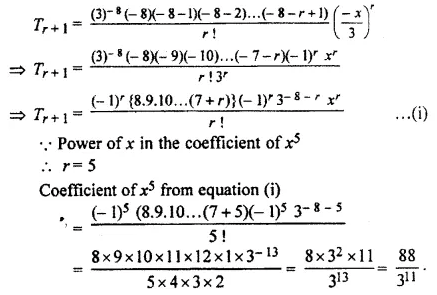Question 5: Find the coefficient of x6 in the expansion of (a + 2bx2)-3.

Solution:

(r + 1)th term in the expansion of (a + 2bx2)-3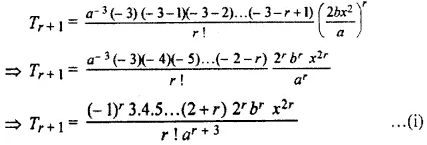For the coefficient of x6 in the this term 2r = 6 ⇒ r = 3

Hence, from equation (i), coefficient of x6 in the expansion of (a + 2bx2)-3

= [(-1)3 3 * 4 * 5 * 23 * b3] / [3! A3+3]

= [-3 * 4 * 5 * 8 * b3] / [3 * 2 * a6]

= [-80b3 / a6]

= -80 b3a-6

Question 6: Find the coefficient of x10 in the expansion of [1 + 3x2] / [1 – x2]3.

Solution: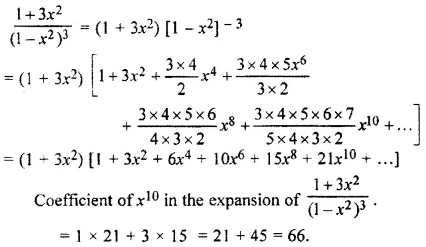Question 7: Find the coefficient of xr in the expression of (1 – 2x + 3x2 – 4x3 + …)n and if x = ½ and n = 1, then find the value of the expression.

Solution:

We know that (1 + x)-2 = 1 – 2x + 3x2 – 4x3 +…+ (-1)r (r + 1)xr + ….. (i)

Given progression (1 – 2x + 3x2 – 4x3 + …)n

= {(1 + x)-2}n [From equation (i)]

= (1 + x)-2n

The (r + 1)th term is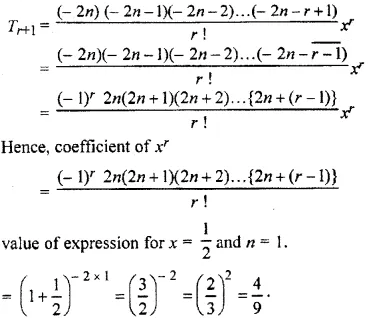Question 8: Prove that (1 + x + x2 + x3 + …)2 = 1 + 2x + 3x2 + …

Solution:

We know that 1 + x + x2 + x3 + … = (1 – x)-1 …. (i)

(1 + x + x2 + x3 + …)2 = {(1 – x)-1}2 [From equation (i)] = (1 – x)2

= (1 – x)2

= 1 + 2x + [2(2 + 1)x2 / 2!] + …….

= 1 + 2x + 3x2 + ……

Question 9: Prove that (1 + x + x2 + x3 +….) (1 + 3x + 6x2 + …) = (1 + 2x + 3x2 + …)2

Solution:

We know that (1 – x)-1 = 1 + x + x2 + x3 +… … (i) and (1 – x)-3 = 1 + 3x + 6x2 + 10x3 + … (ii) L.H.S.

= (1 + x + x2 + x3 +…) (1 + 3x + 6x2 + …)

= {(1 – x)-1} {(1 – x)-3}

= {(1 – x)2}-2

= {(1 – x)-2}2

= (1 + 2x + 3x2 +…)2

= R.H.S.

Question 10: If x = 2y + 3y2 + 4y3 + …then express y in a series of ascending powers of x.

Solution:

⇒ x = 2y + 3y2 + 4y2 + …

⇒ x = (1 + 2y + 3y2 + 4y3 + …)-1 [∵ (1 – x)-2 = 1 + 2x + 3x2 + …]

⇒ (x + 1) = (1 – y)-2

(1 – y2) = 1 / (1 + x)

(1 – y2) = (1 + x)-1

Taking square root on both sides,

(1 – y) = (1 + x)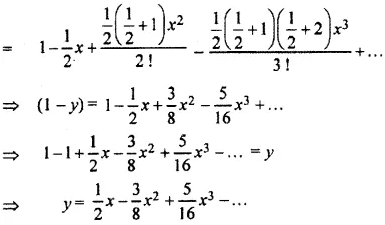### RBSE Maths Chapter 8: Exercise 8.5 Textbook Important Questions and Solutions

Question 1: If y is too small as compared to x, then prove that [x – y]n / [x + y]n = 1 – [2ny / x], where y2 and higher power are negligible.

Solution: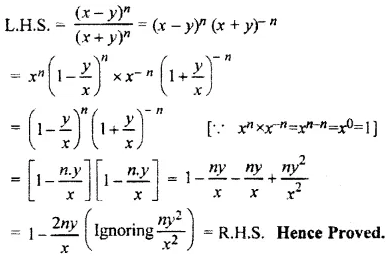Question 2: If x is too small such that x2 and higher powers are negligible then find the value of the expression {[9 + 2x]½ (3 + 4x)} / [1 + x].

Solution:

{[9 + 2x]½ (3 + 4x)} / [1 + x]

= {½ [1 + (2x / 9)]½ [3 + 4x]} / [1 + x]

= (3 [1 + ½ * [2x / 9] + {[(½) (½ – 1) * [(2 / 9) * x]2} / 2! + ….. ] * [3 + 4x]) / [1 + (1 / 5) * x + ([1 / 5] – 1) (x2 / 2!)]

Ignoring x2 and terms of higher order,

= {3[1 + (x / 9)] [3 + 4x]} / [1 + (x / 5)]

= [5(9 + x) (3 + 4x)] / [3(5 + x)]

= {5(27 + 3x + 36x + 4x2)} / {3(5 + x)}

= 5(9 + 3x) / (5 + x)

= (45 + 65x) (5 + x)-1

= 9 + 13x – (9x / 5) – (13x2 / 5)

= 9 + (56 / 5)x

Question 3: Find the value of

[i] √30 up to 4 decimal places.

[ii] (1.03) up to 4 decimal places

Solution:

[i] √30 up to 4 decimal places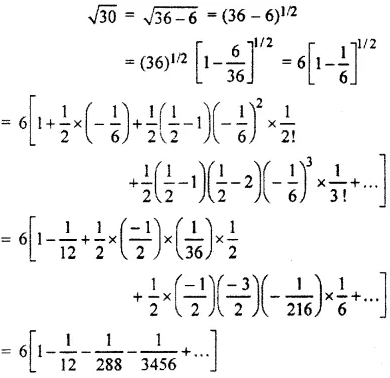= 6 (1 – 0.08333 – 0.00347 – 0.00029 + …]

= 6 (0.9122)

= 5.47746

(ii) (1.03) up to 4 decimal places

(1.03) = (1 + 0.03)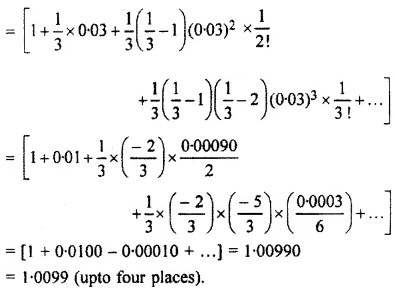Question 4: If x is approximately equal to 1, then prove that

[i] [mxm – nxn] / [m – n] = xm+n

[ii] [axb – bxa] / [xb – xa] =  / [1 – x]

Solution:

[i] [mxm – nxn] / [m – n] = xm+n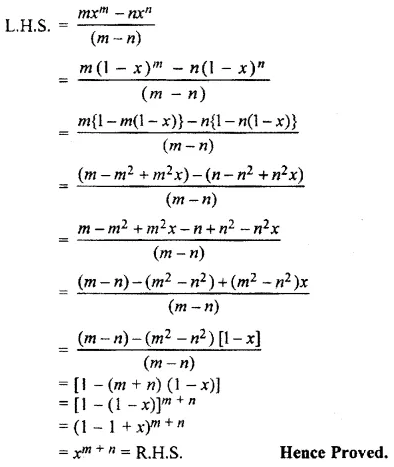[ii] [axb – bxa] / [xb – xa] =  / [1 – x]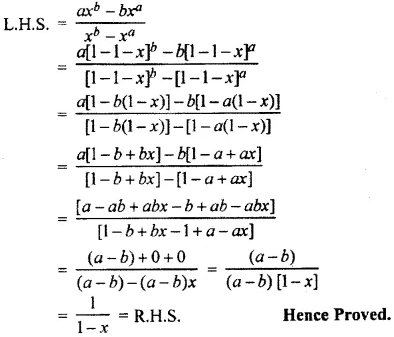### RBSE Maths Chapter 8: Exercise 8.6 Textbook Important Questions and Solutions

Question 1: 1 + [2 / 3] * [1 / 2] + {[2 * 5] / [3 * 6]} * [1 / 22] + ……..

Solution:

On comparing the given series with 1 + nx + [{n (n – 1) x2} / 2!] + ……..

nx = [2 / 3] * [1 / 2]

nx = [1 / 3]

n2x2 = 1 / 9

1 / [n2x2] = 9 —- (1)

[{n (n – 1) x2} / 2!] = {[2 * 5] / [3 * 6]} * [1 / 22] [n(n – 1) / 2!] * x2 = 5 / [9 * 22] —- (2)

Multiply equation (1) and (2),

[n – 1] / 2n = 5 / 4

4n – 4 = 10n

6n = -4

n = -2 / 3

If n = [-2 / 3], then [-2 / 3] * x = [1 / 3]

x = [-1 / 2]

Hence the sum of the given series,

= (1 + x)n

= (1 – (1 / 2))-⅔

= (1 / 2)-⅔

= (22)

= (4)

Question 2: 1 + [1 / 3] * [1 / 4] + {[(1 * 4) / (3 * 6)] * [1 / 42]} + ……

Solution:

On comparing the given series with the given series,

1 + nx + [n(n – 1) / 2!] * [x2] + ……

nx = 1 / 12

[1 / n2x2] = 144 —- (1)

[n(n – 1) x2 / 2] = [1 / 72] —- (2)

Multiply equation (1) and (2)

[n(n – 1) x2 / 2] * [1 / n2x2] = [1 / 72] * 144

(n – 1) / 2n = 2

n – 1 = 4n

n = [-1 / 3]

From equation (1),

[-1 / 3] * x = [1 / 12]

x = [-1 / 4]

The sum of given series,

(1 + x)n = (1 – (1 / 4))-⅓

= (3 / 4)-⅓

= (4 / 3)